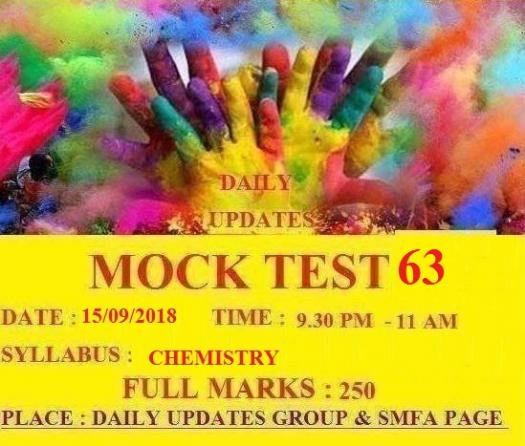# Daily Updates Mock Test 63

50 Questions | Total Attempts: 152Settings• 1.
The most abundant rare gas in the atmosphere is
• A.

He

• B.

Ne

• C.

Ar

• D.

Xe

• 2.
The Latin word formica means ant. The name formic acid is derived from this Latin word because
• A.

This acid, in ancient times, was used to eliminate ant-hills

• B.

This corrosive acid is secreted by ants to drive away their enemies

• C.

This acid was first obtained by the distillation of ants

• D.

Ants are attracted by the odour of this acid

• 3.
The ore which is found in abundance in India is
• A.

Monazite

• B.

Fluorspar

• C.

Bauxite

• D.

Magnetite

• 4.
The inherited traits of an organism are controlled by
• A.

RNA molecules

• B.

Nucleotides

• C.

DNA molecules

• D.

Enzymes

• 5.
The heat energy produced when the human body metabolises 1 gram of fat is
• A.

30 KJ

• B.

1 KJ

• C.

39 KJ

• D.

29 KJ

• 6.
What are the number of moles of CO2 which contains 16 g of oxygen?
• A.

0.5 mole

• B.

0.2 mole

• C.

0.4 mole

• D.

0.25 mole

• 7.
The main use of salt in the diet is to
• A.

Make the taste of food better

• B.

Produce in small amounts the hydrochloric acid required for the digestion of food

• C.

Ease the process of cooking

• D.

Increase the solubility of food particles in water

• 8.
The monomer of polythene is
• A.

Vinyl chloride

• B.

Ethylene

• C.

Ethyl alcohol

• D.

None of the above

• 9.
The luster of a metal is due to
• A.

its high density

• B.

Its high polishing

• C.

Its chemical inertness

• D.

Presence of free electrons

• 10.
The number of water molecules present in a drop of water (volume 0.0018 ml) at room temperature is
• A.

1.568 x 103

• B.

6.023 x 1019

• C.

4.84 x 1017

• D.

6.023 x 1023

• 11.
The most malleable metal is
• A.

Platinum

• B.

Silver

• C.

Iron

• D.

Gold

• 12.
The oil used in the froth floatation process is
• A.

Coconut oil

• B.

Olive oil

• C.

Kerosene oil

• D.

Pine oil

• 13.
The number of waves in n x 10th Bohr's orbit are
• A.

N2

• B.

N

• C.

N-2

• D.

N3

• 14.
The mass of one Avogadro number of helium atom is
• A.

1.00 gram

• B.

4.00 gram

• C.

8.00 gram

• D.

4 x 6.02 x 1023 gram

• 15.
The items amenable to detection by soft x-rays are
• A.

Contrabands

• B.

• C.

Narcotics

• D.

Genuine coins from counterfeit coins

• 16.
The material which can be deformed permanently by heat and pressure is called a
• A.

Thermoplastic

• B.

Thermoset

• C.

Chemical compound

• D.

Polymer

• 17.
The mass number of a nucleus is
• A.

Always less than its atomic number

• B.

The sum of the number of protons and neutrons present in the nucleus

• C.

Always more than the atomic weight

• D.

A fraction

• 18.
The inexpensive and commonly used variety of glass is called soda glass. It is called so because
• A.

Was used initially for making bottles of soda(carbonated drink)

• B.

• C.

Was initially used for storing sodium carbonate

• D.

• 19.
The gas used in the manufacture of vanaspati from vegetable oil is
• A.

Hydrogen

• B.

Oxygen

• C.

Nitrogen

• D.

Carbon dioxide

• 20.
The ionic radii of N3-, O2-, F- and Na+ follows the order
• A.

N3- > O2- > F- > Na+

• B.

N3- > Na+ > O2- > F-

• C.

Na+ > O2- > N3- > F-

• D.

O2- > F- > Na+ > N3-

• 21.
The graphite rods in the nuclear reactor
• A.

React with U to release energy

• B.

Produce neutrons

• C.

Undergo combustion which triggers the nuclear fission

• D.

Convert fast moving neutrons into thermal neutrons

• 22.
The first metal used by man was
• A.

Iron

• B.

Copper

• C.

Gold

• D.

Bronze

• 23.
The hydronium ion is
• A.

H+

• B.

HO -

• C.

H2+

• D.

H3O+

• 24.
The most electropositive elements among the following is
• A.

Na

• B.

Ca

• C.

K

• D.

Cs

• 25.
The method that cannot be used for removing permanent hardness of water is
• A.

• B.

Distillation

• C.Back to top